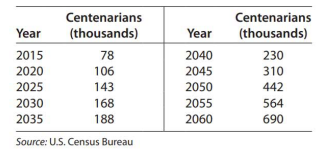# Modeling Centenarians The following table gives the projected population, in thousands, of Americans over 100 years of age. Create an exponential function that models the projected population y, in thousands, as a function of the number of years past 2010. Report the model with three significant digits. Use the reported model to find a function that gives the rate of change in the projected number of centenarians. What is the projected rate of change in the number of centenarians in 2040?### Mathematical Applications for the ...

11th Edition
Ronald J. Harshbarger + 1 other
Publisher: Cengage Learning
ISBN: 9781305108042

#### Solutions

Chapter
Section### Mathematical Applications for the ...

11th Edition
Ronald J. Harshbarger + 1 other
Publisher: Cengage Learning
ISBN: 9781305108042
Chapter 11.2, Problem 64E
Textbook Problem
1 views

## Modeling Centenarians The following table gives the projected population, in thousands, of Americans over 100 years of age. Create an exponential function that models the projected population y, in thousands, as a function of the number of years past 2010. Report the model with three significant digits. Use the reported model to find a function that gives the rate of change in the projected number of centenarians. What is the projected rate of change in the number of centenarians in 2040?This textbook solution is under construction.

### Still sussing out bartleby?

Check out a sample textbook solution.

See a sample solution

#### The Solution to Your Study Problems

Bartleby provides explanations to thousands of textbook problems written by our experts, many with advanced degrees!

Get Started